Types of Quadrilaterals – Rectangle, Square, Rhombus, Parallelogram | Class 8 Maths

• Last Updated : 27 Oct, 2020

Quadrilaterals can be defined as, types of polygons that have four sides, four vertices, and four angles along with a pair of diagonals. The Sum of interior angles of quadrilaterals is 360°. There are various kinds of quadrilaterals. As the name itself suggest the word is a combination of two Latin words ‘Quadri‘ means a variant of four, and ‘latus‘ means side.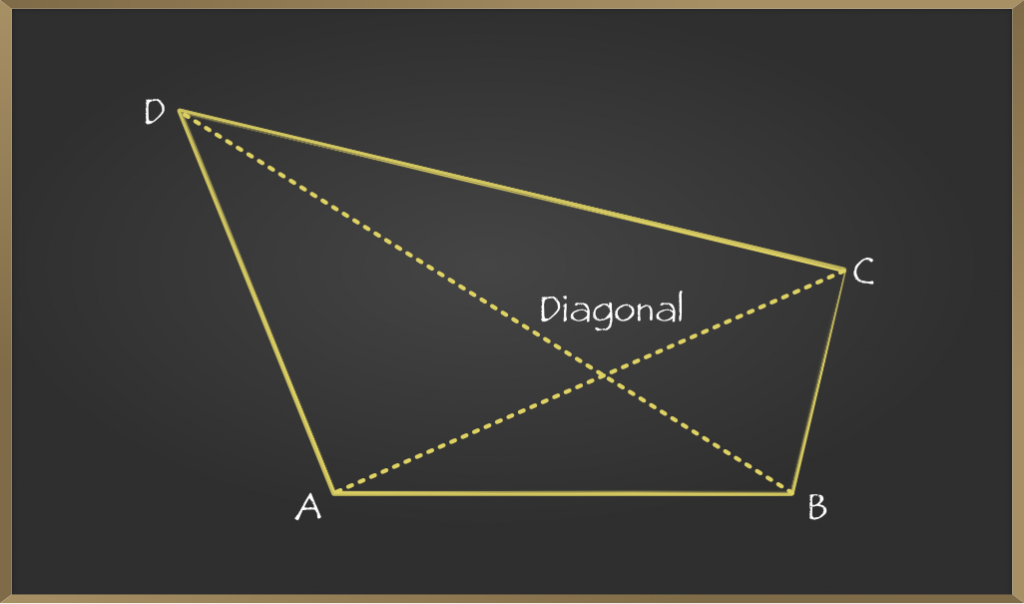Quadrilaterals based on their properties can be subdivided into other groups and the main subdivision of the quadrilaterals is between convex and concave quadrilaterals. These concave and convex quadrilaterals can be further classified into their subdivision.

Quadrilaterals which have one of the interior angle greater than 180° and one diagonal lies outside the quadrilateral are called convex quadrilaterals.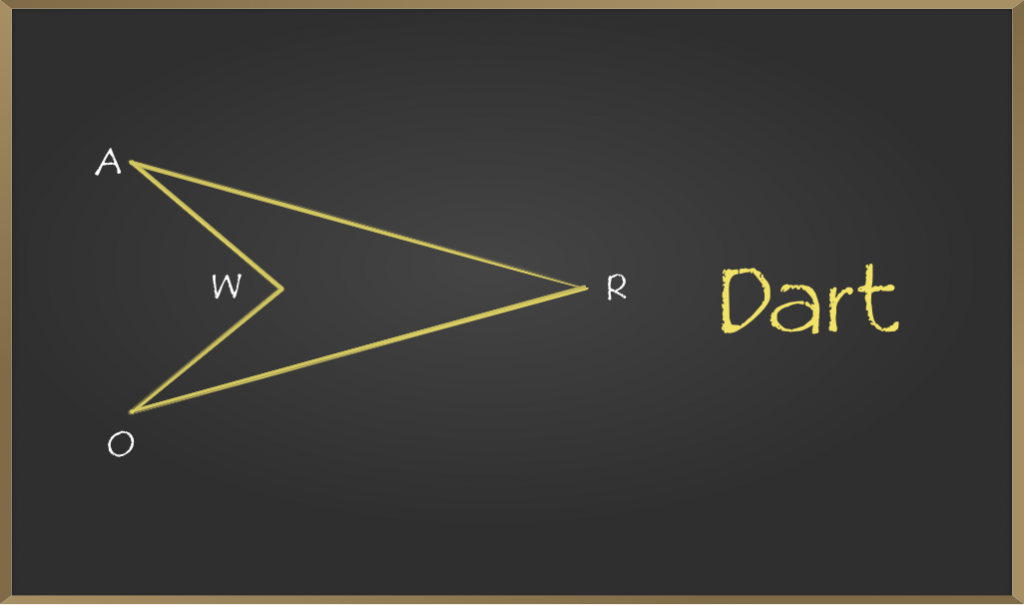One of the examples of a concave quadrilateral is a Dart. It is a quadrilateral with bilateral symmetry like a kite, but with a reflex interior angle.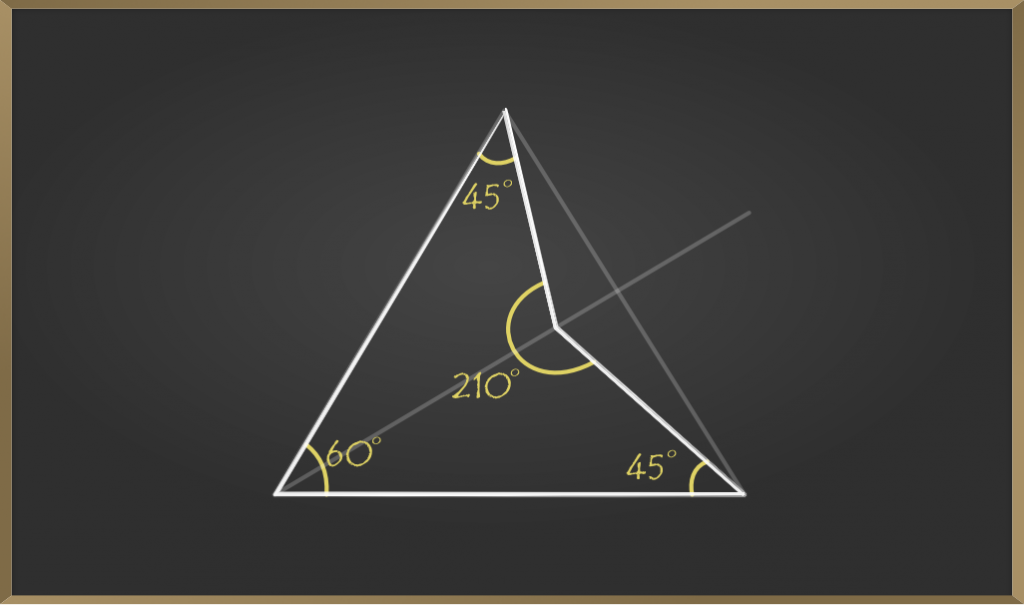Quadrilaterals which have all four interior angle less than 180° are called concave quadrilaterals. There are various types of Convex Quadrilaterals:

• Trapezium
• Kite
• Parallelogram
• Rectangle
• Rhombus
• Square

Trapezium

A trapezium is a quadrilateral which has one pair of opposite sides parallel. In a regular trapezium, non-parallel sides are equal and its base angles are equal.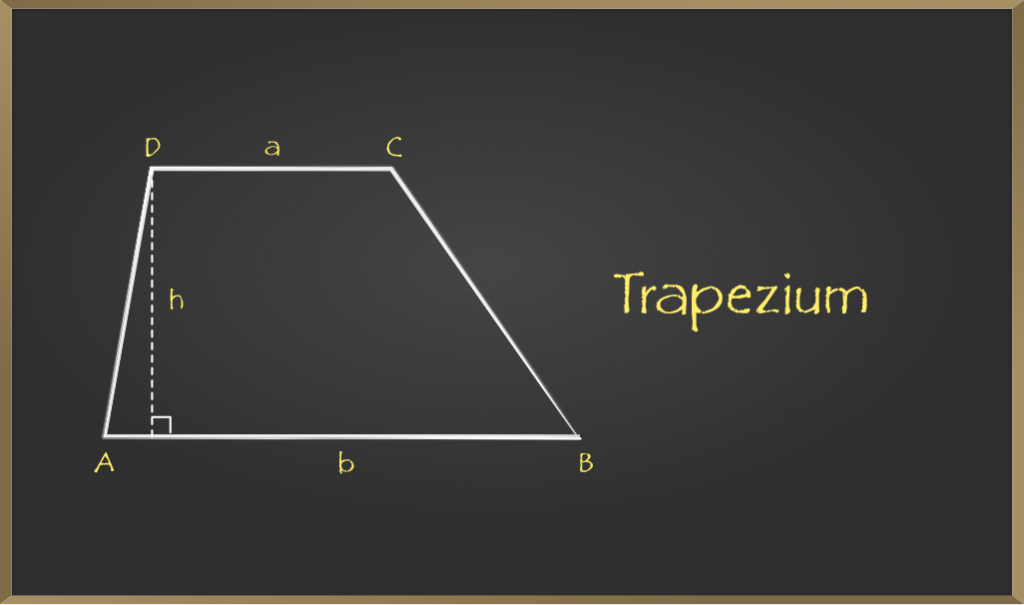Kite

Kite has two pairs of equal adjacent sides and one pair of opposite angles equal. Diagonals of kite intersect perpendicularly. The longest diagonal of the kite bisects the smaller one.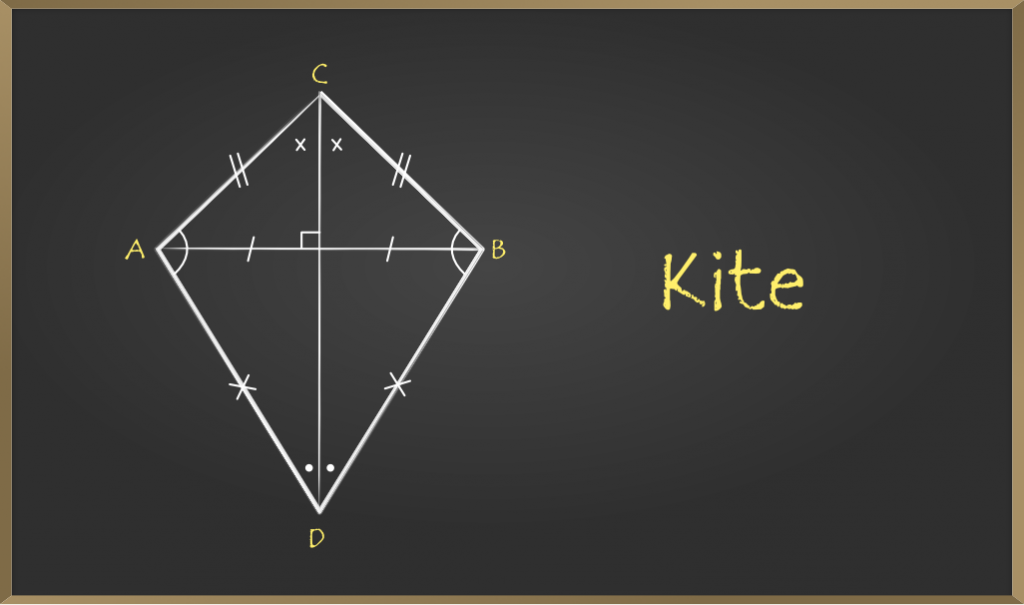Parallelogram

A quadrilateral whose opposite sides are equal and parallel is called a Parallelogram. Opposite angles of a Parallelogram are equal and its diagonals bisect each other.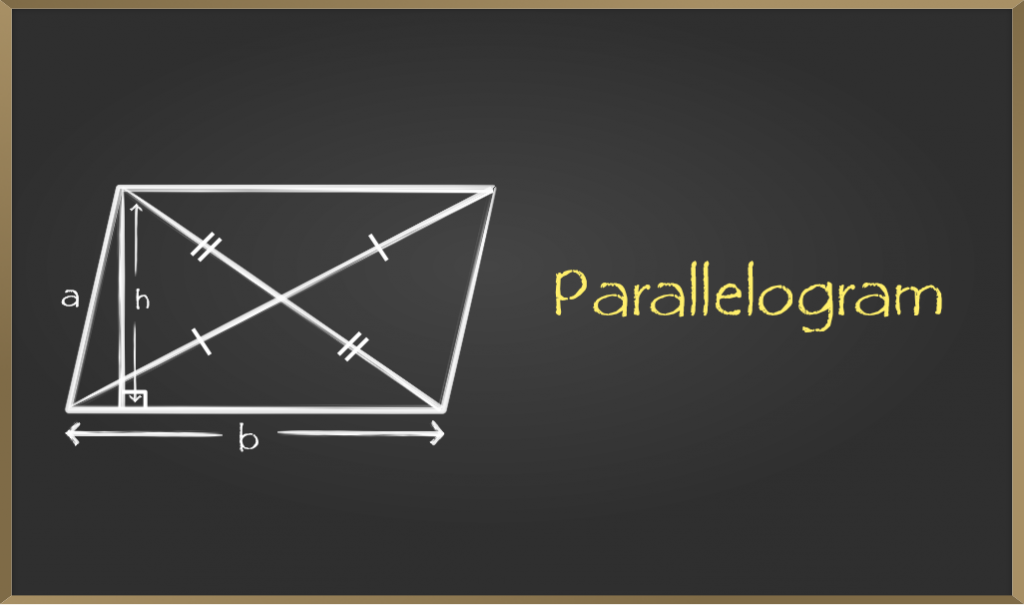Rectangle

A quadrilateral whose opposite sides are equal and parallel and all the interior angles equal to 90° is defined as Rectangle. Diagonals of rectangle bisect each other. Note that all the rectangles are parallelograms but the reverse of this is not true.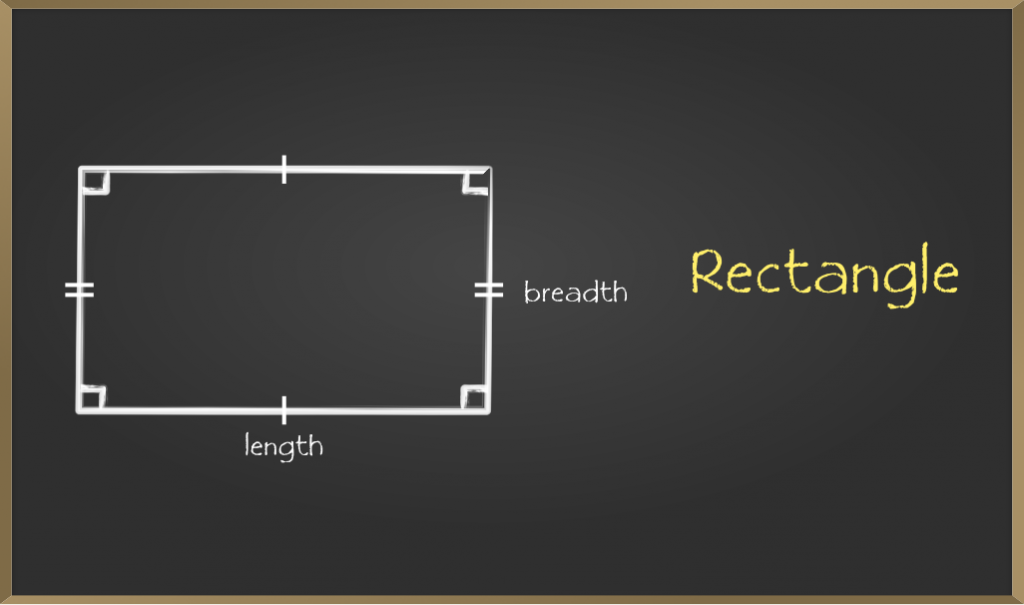Rhombus

A quadrilateral that has all sides equal and opposite sides parallel is called Rhombus. Opposite angles of a rhombus are equal and diagonals of the Rhombus bisect each other perpendicularly. Note all rhombus are parallelograms but the reverse of this is not true.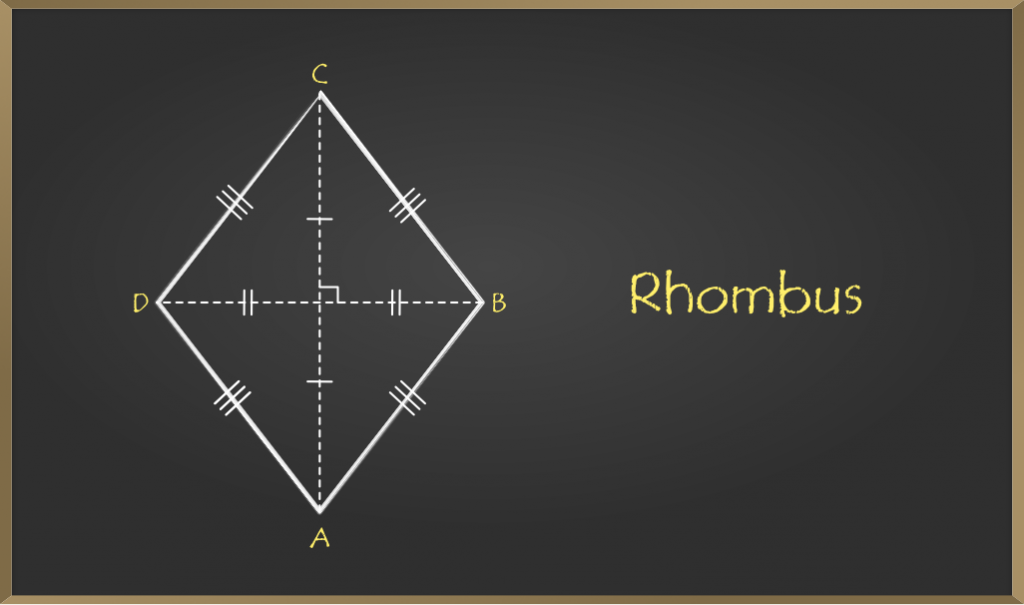Square

A quadrilateral that has all sides equal and opposite sides parallel and all interior angles equal to 90° is called Square. Diagonals of square bisect each other perpendicularly. Note that all squares are rhombus but not vise-versa.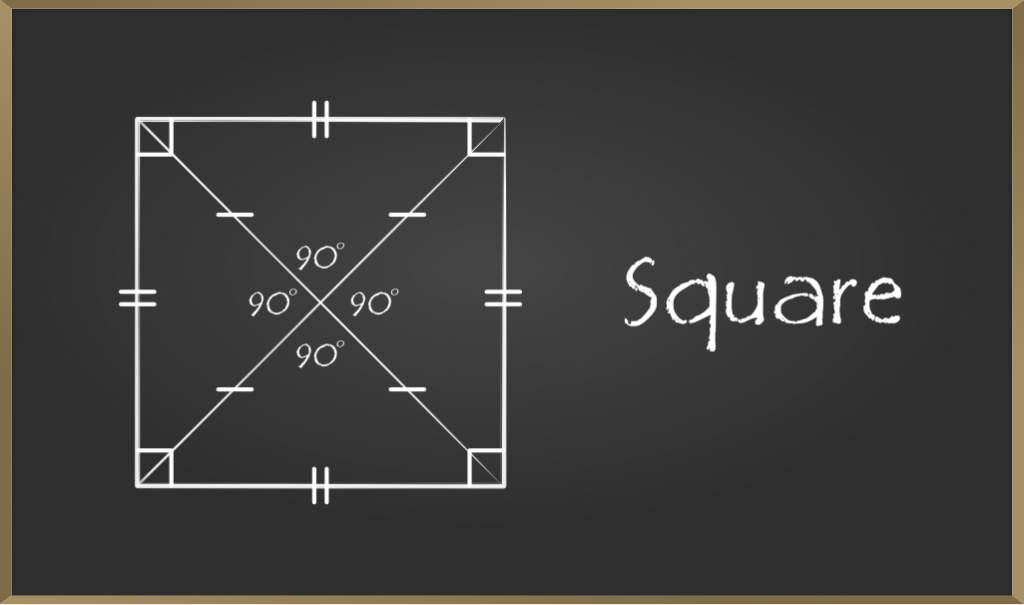Now let us see some problems based on quadrilaterals :

Problem 1: Perimeter of quadrilateral ABCD is 46 units. AB = x + 7, BC = 2x + 3, CD = 3x – 8, and DA = 4x – 6. Find the length of the shortest side of the quadrilateral.

Solution

Perimeter = Sum of all sides

=> 46 = 10x – 4 or [x = 5]

That gives, AB = 12 units, BC = 13 units, CD = 7 units, DC = 14 units

Hence length of shortest side is 7 units (i.e. CD).

Problem 2: Given a trapezoid ABCD (AB || DC) with median EF. AB = 3x – 5, CD = 2x -1 and EF = 2x + 1. Find value of EF.

Solution

We know that the Median of the trapezoid is half the sum of its bases.

=> EF = (AB + CD) / 2

=> 4x + 2 = 5x – 6  or [ x = 8 ]

Therefore EF = 2x + 1 = 2(8) + 1 => EF = 17 units.

Problem 3: In a Parallelogram adjacent angles are in the ratio of 1:2. Find the measures of all angles of this Parallelogram.

Solution

Let the adjacent angle be x and 2x.

We know that in of a Parallelogram adjacent angles are supplementary.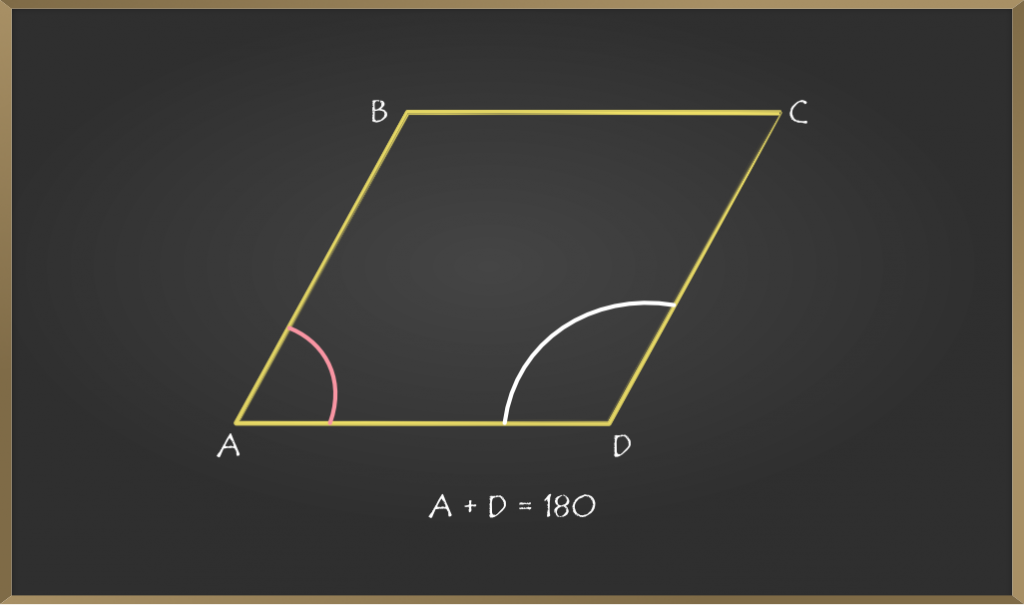=> x + 2x = 180° or [ x = 60° ]

Also, opposite angles are equal in a Parallelogram.

Therefore measures of each angles are 60°, 120°, 60°, 120°.

Problem 4: Perimeter of a rhombus is 52 units and the length of one of its diagonal is 24 units. Find the length of another diagonal.

Solution:

Given: Perimeter = 52 unit.

Length of diagonal (say AC) = 24 Units.

We know that, rhombus has its all four sides equal.

=> AB + BC + CD + DA = 52.

=> 4.(AB) = 52 => AB = 13 units.

Also, diagonals of Rhombus bisects each other perpendicularly. Hence in given picture AE = EC and BE = ED and ∠AEB = 90°.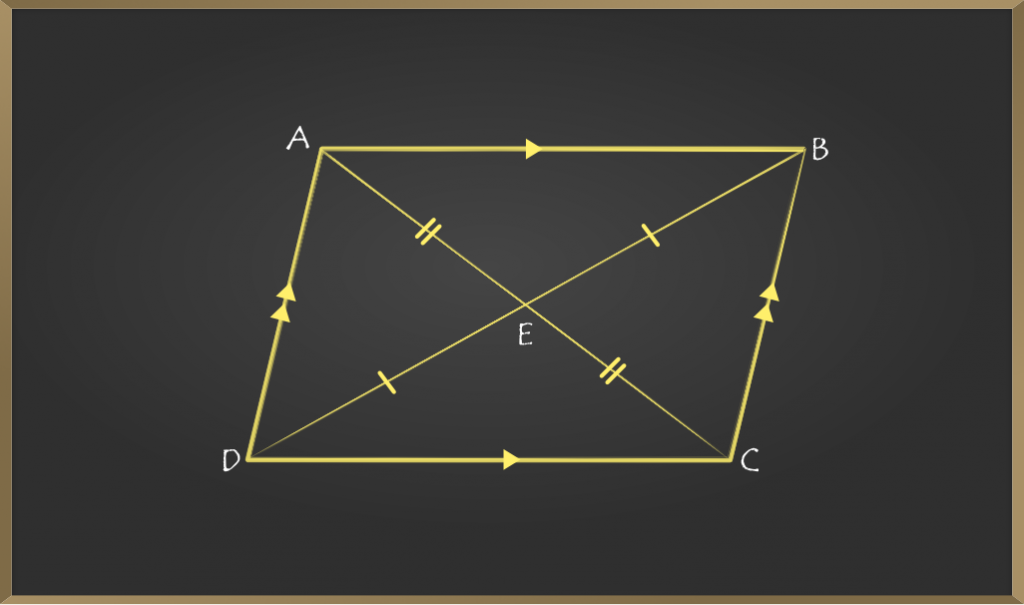Applying Pythagoras theorem in ∆AEB,(∠AEB = 90°)

=> (AB)² = (AE)² + (EB)²

=> (13)² = (12)² + (EB)²

=> (EB)² = 169 – 144 = 25

=>   EB  =  5 units.

Since, AC = 2*EB = 10 units.

Therefore, required length of another diagonal of rhombus is 10 units.

My Personal Notes arrow_drop_up